# Blinn-Phong 着色模型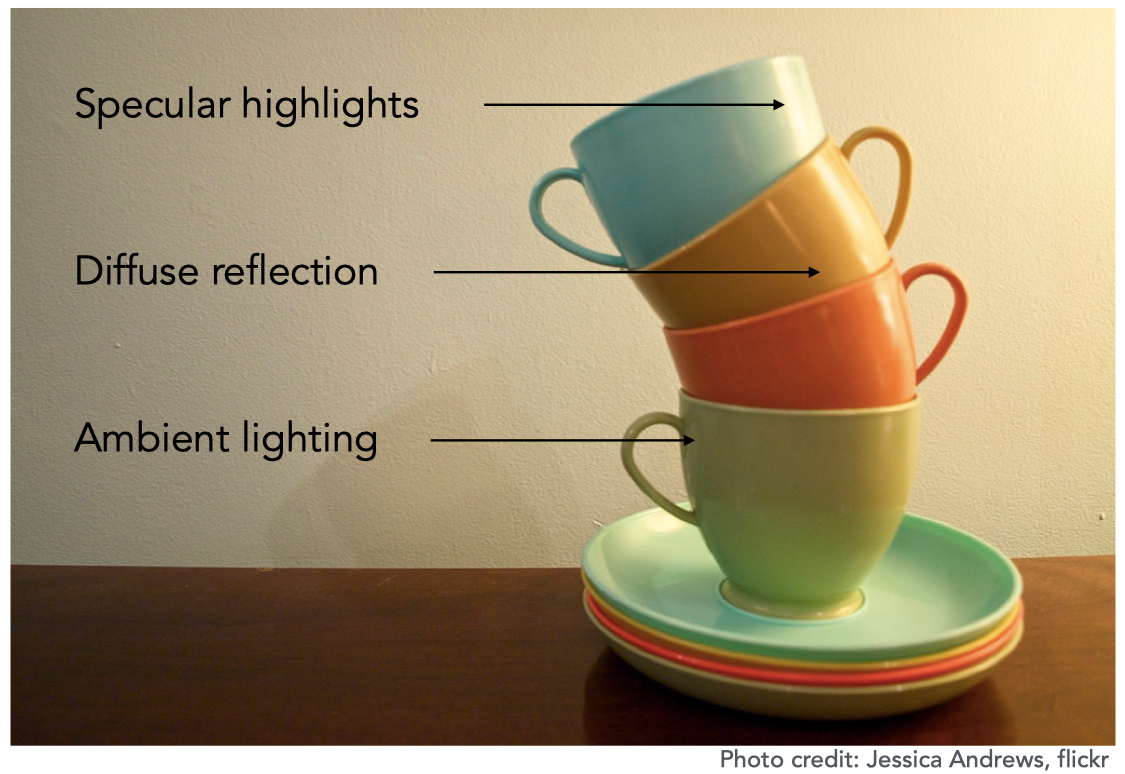Blinn-Phong 着色模型主要有三部分组成：

• Diffuse （漫反射）：由物体本身的颜色决定
• Specular （高光）：由观察角度与光线反射角度共同决定，两者夹角越小，高光越明显
• Ambient （环境光）：由环境决定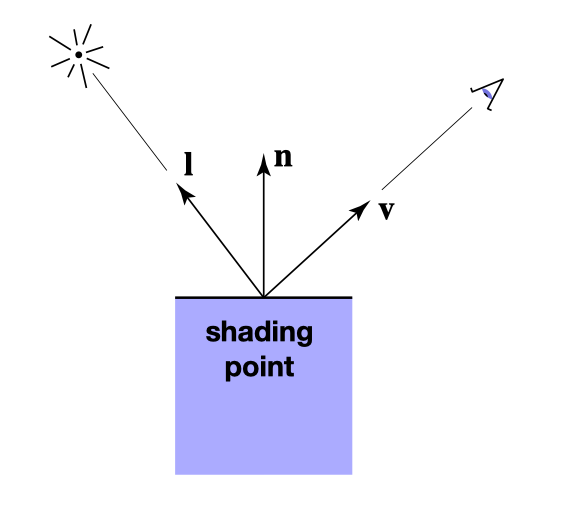• n 表示表面法线方向
• l 表示入射光方向
• v 表示观察方向

## 漫反射 （Diffuse）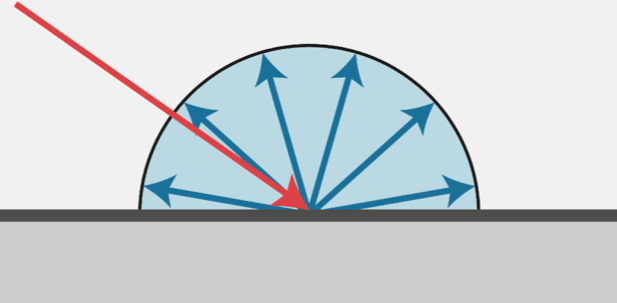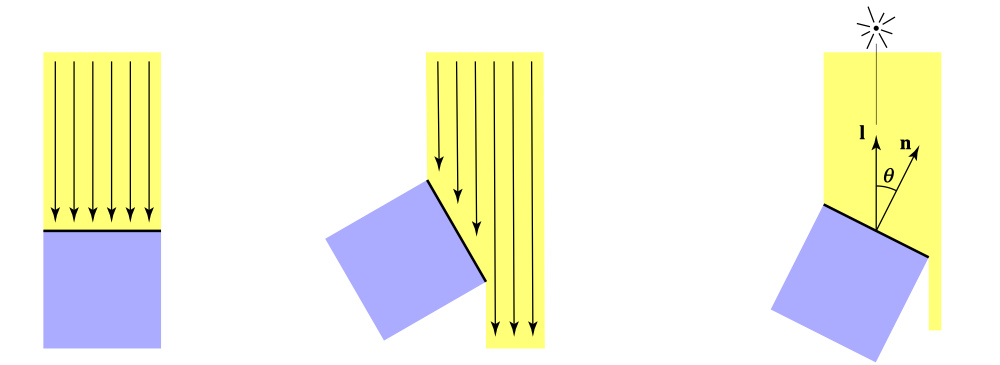$$平面接受的能量的百分比 = cos(n, l) = n \cdot l$$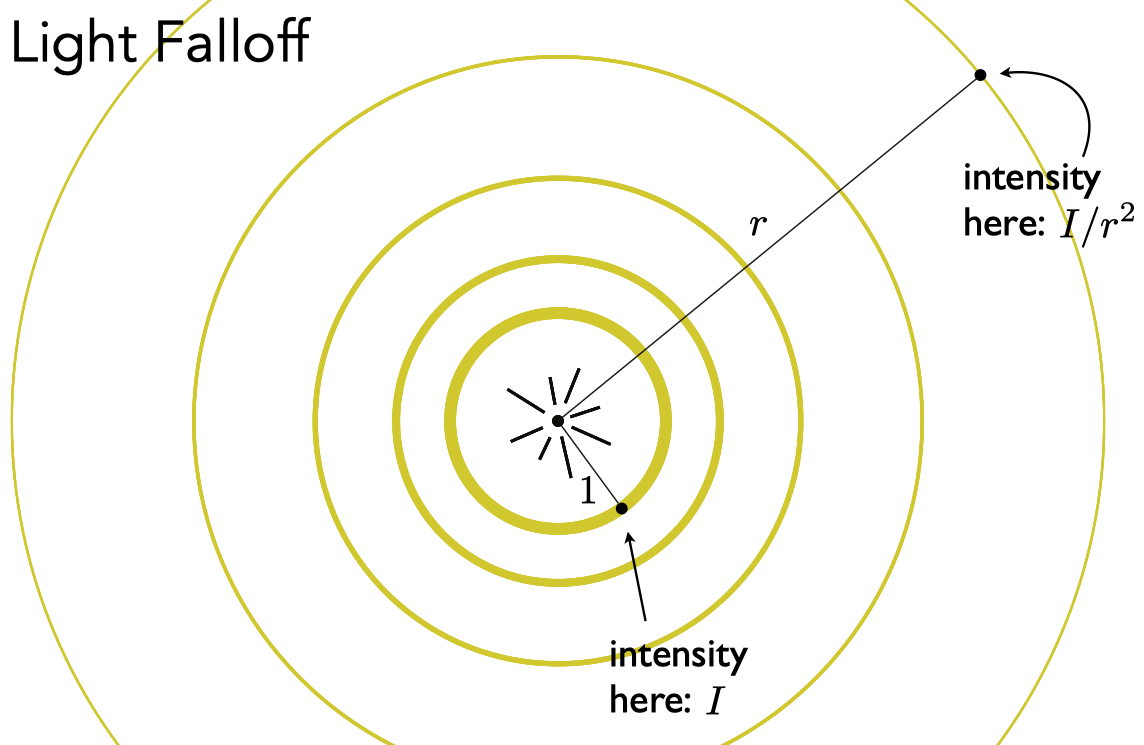$$S = 4 \pi r^2$$

$$E = I_{1} \times 4 \pi$$

$$E = I_{r} \times 4 \pi r^2$$

$$I_{r} = \frac {I_1} {r^2}$$

$$到达着色点的能量 = \frac {I} {r^2}$$

$$被着色点吸收的能量的百分比 = max(0, n \cdot l)$$

$$L_d = k_d \cdot (\frac I {r^2}) \cdot max(0, n \cdot l)$$

• $L_d$ 表示着色点的漫反射分量
• $k_d$ 表示材质的漫反射系数，可调节

## 高光（Specular）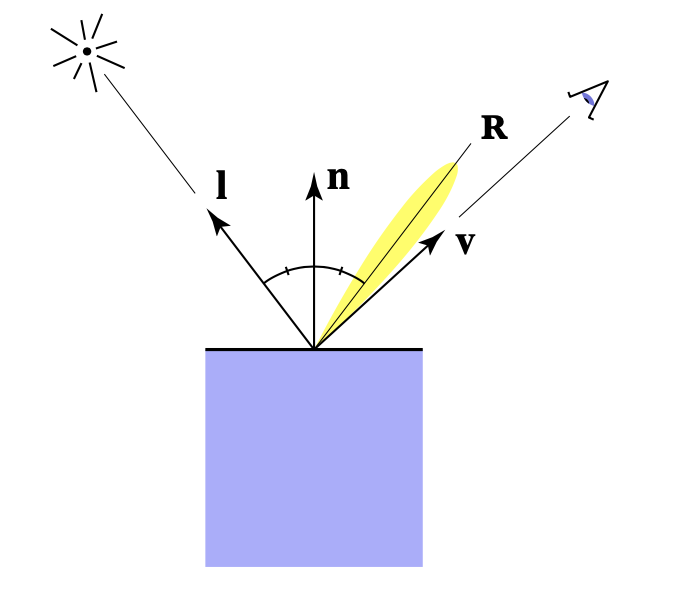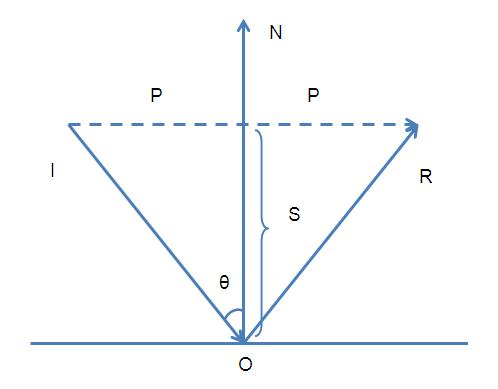$$\vec {OR} = \vec {IR} - \vec {IO}$$

$$\vec {OR} = 2 \vec {P} - \vec {IO}$$

$$\vec {P} = \vec {IO} + \vec {S}$$

$$\vec {S} = \vec {ON} (-\vec {IO} \cdot \theta) = -\vec {ON} (\vec {IO} \cdot \vec {ON})$$

$$\vec {OR} = 2(\vec {IO} - \vec {ON} (\vec {IO} \cdot \vec {ON})) - \vec {IO}$$

$$\vec {OR} = \vec {IO} - 2 \vec {ON} (\vec {IO} \cdot \vec {ON}))$$

$$\vec r = 2 \vec n (\vec l \cdot \vec n) - \vec l$$

$$L_s = k_s \cdot (\frac I {r^2}) \cdot max(0, v \cdot r)$$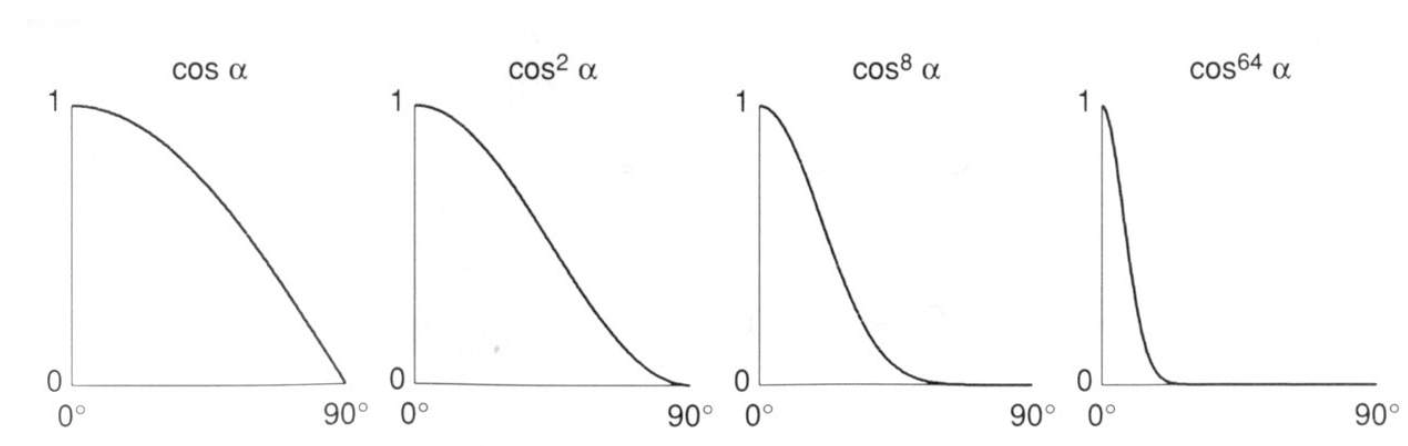$$L_s = k_s \cdot (\frac I {r^2}) \cdot max(0, v \cdot r)^p$$

• $L_s$ 表示着色点的高光分量
• $k_s$ 表示材质的高光系数，可调节
• $p$ 用来调节高光的可视范围

Blinn Phong 着色模型在这基础上进行了优化：当反射向量与视角向量相近时，这相当于视角与入射光的 半程向量 与法线的夹角相近：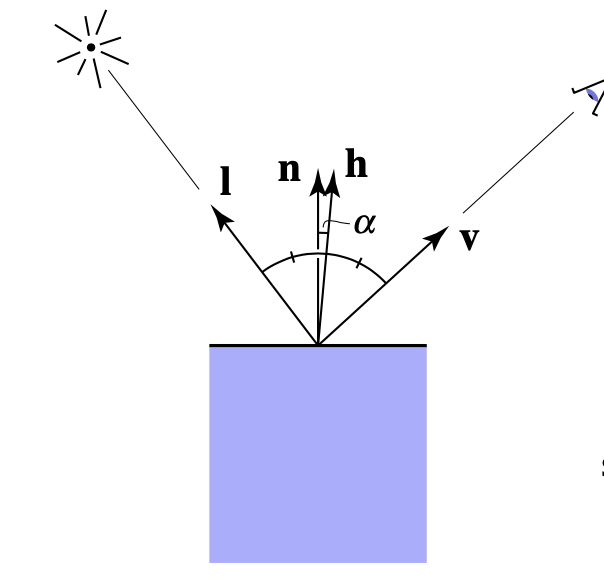$$\vec h = \frac {\vec l + \vec v} {||\vec l + \vec v||}$$

$$L_s = k_s \cdot (\frac I {r^2}) \cdot max(0, n \cdot h)^p$$

## 环境光（Ambient）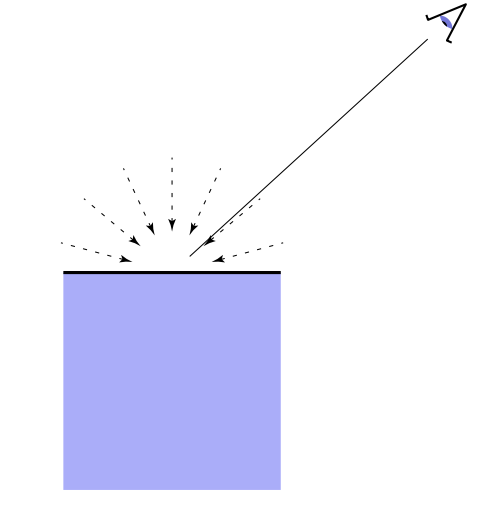$$L_a = k_a I_a$$

• $L_a$ 表示着色点的环境光分量
• $k_a$ 表示材质的环境光系数，可调节
• $I_a$ 表示场景的环境光强度Blinn Phong 的着色公式为：

$$L = L_a + L_d + L_s$$ $$L = k_a I_a + k_d(\frac {I} {r^2}) max(0, n \cdot l) + k_s(\frac {I} {r^2}) max(0, n \cdot h)^p$$

# 着色频率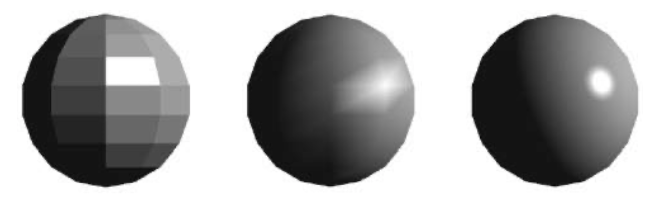## 对比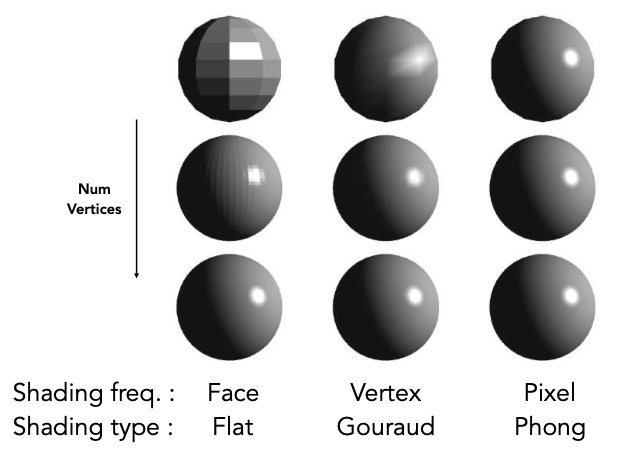# 纹理映射（Texture Mapping）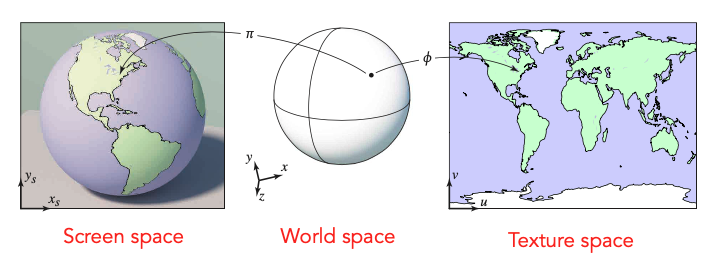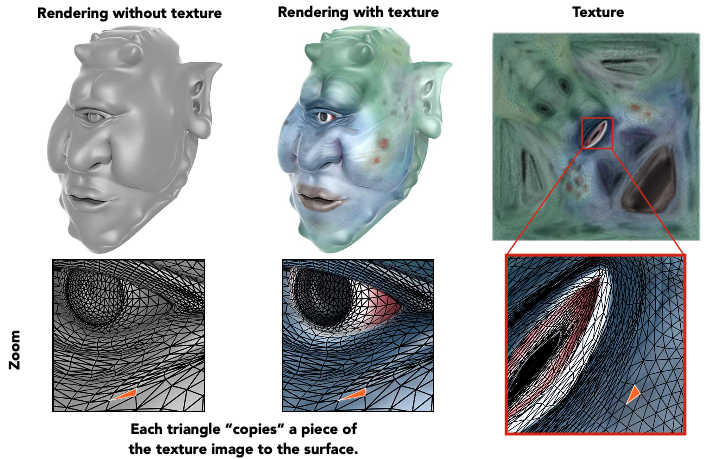UV 坐标是指以纹理左下角为原点来建立的二维坐标系，横坐标为 U，纵坐标为 V，它们的取值范围都是 $[0, 1]$，这个取值范围与贴图尺寸无关，即纹理最大宽高在 UV 中都是表示为 1。# 重心坐标（Barycentric Coordinates）$$p = a + \beta(b - a) + \gamma(c - a)$$

$$p = (1 - \beta - \gamma)a + \beta b + \gamma c$$

$$p = \alpha a + \beta b + \gamma c$$

$$\alpha + \beta + \gamma = 1$$

$$p = (\alpha, \beta, \gamma)$$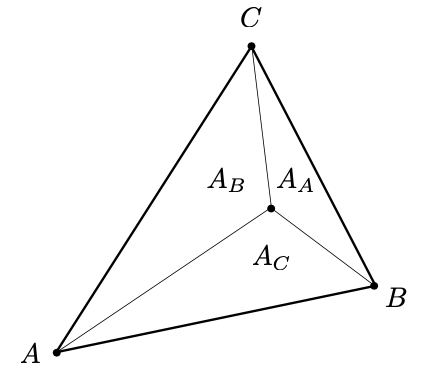$$\alpha = \frac {A_A} {A_A + A_B + A_C}$$ $$\beta = \frac {A_B} {A_A + A_B + A_C}$$ $$\gamma = \frac {A_C} {A_A + A_B + A_C}$$

• $(1, 0, 0)$：顶点 A 的坐标
• $(0, 1, 0)$：顶点 B 的坐标
• $(0, 0, 1)$：顶点 C 的坐标
• $(\frac 1 3, \frac 1 3, \frac 1 3)$：三角形的重心

$$\alpha = \frac {-(x - x_B)(y_C - y_B) + (y - y_B)(x_C - x_B)} {-(x_A - x_B)(y_C - y_B) + (y_A - y_B)(x_C - x_B)}$$ $$\beta = \frac {-(x - x_C)(y_A - y_C) + (y - y_C)(x_A - x_C)} {-(x_B - x_C)(y_A - y_C) + (y_B - y_C)(x_A - x_C)}$$ $$\gamma = 1 - \alpha - \beta$$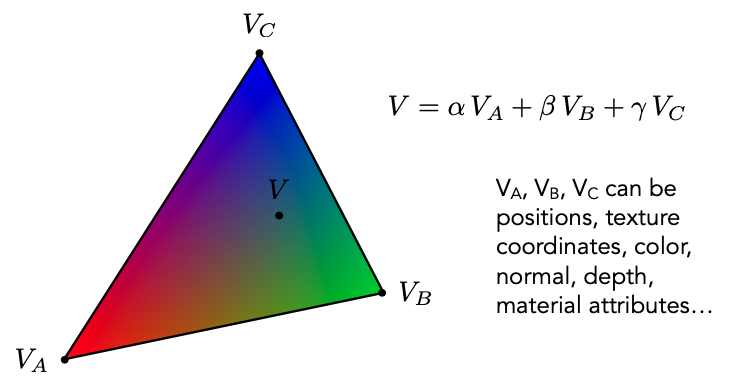# 应用纹理

 1 2 3 4  for each pixel(x, y): uv = getUv(x, y) texColor = sample2D(texture, uv) kd = texColor 

## 纹素（Texel）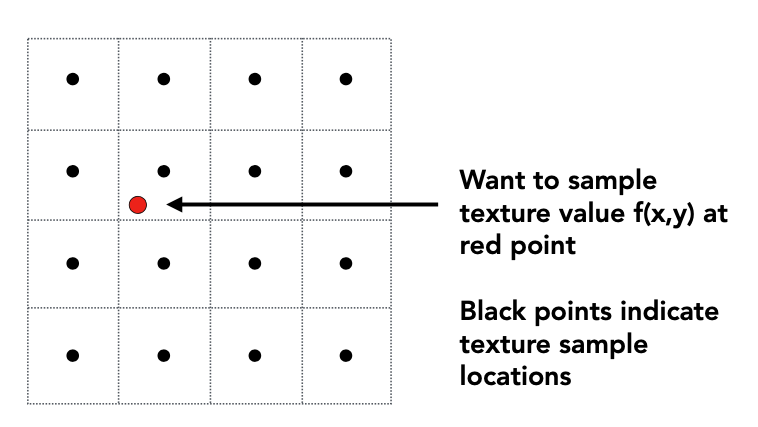• Nearest 取该采样点所在的纹素的颜色（会发生一次采样）

• Linear 取该采样点周围四个纹素的颜色，然后进行加权平均（会发生四次采样）

## 纹理放大（Texture Magnification）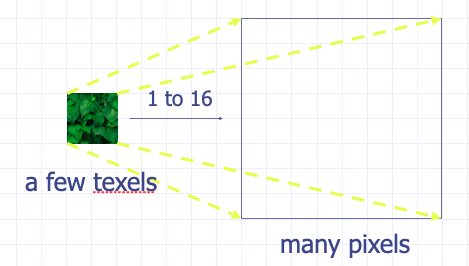## 纹理缩小（Texture Minification）## 实际问题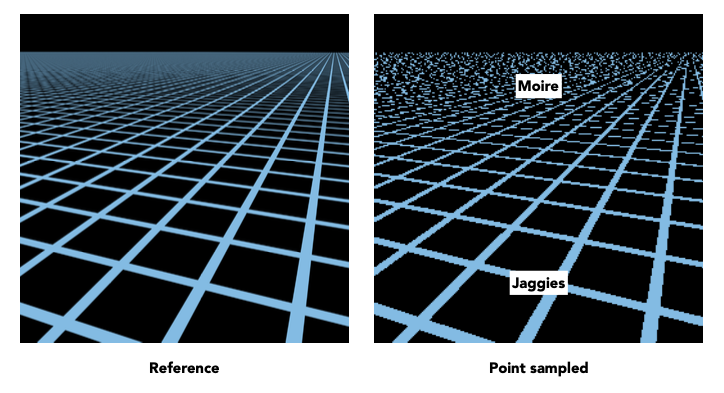• 对于近距离的像素来说，它所覆盖的纹素范围较少，纹理发生了放大。

• 对于远距离的像素来说，它所覆盖的纹理范围较大，纹理发生了缩小。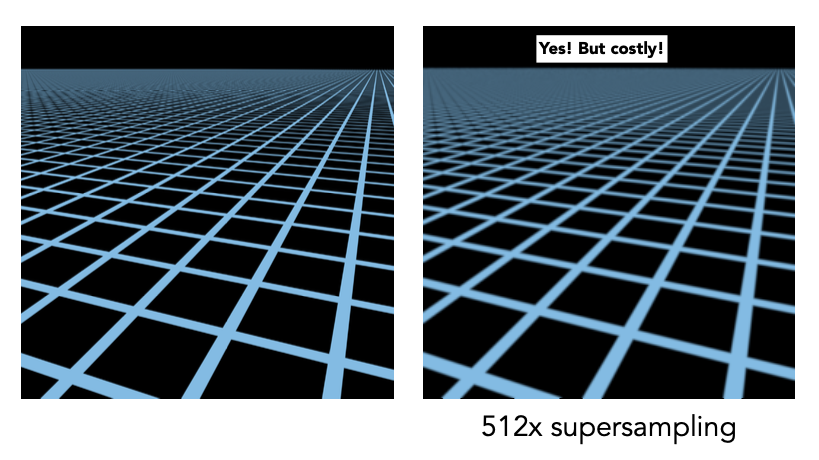## Mipmap

Mipmap 是指根据一张纹理去生成一系列更小的纹理的技术。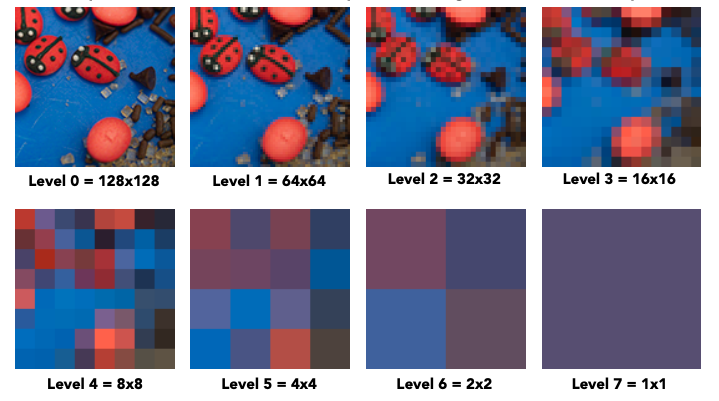• 第 0 级是纹理的原尺寸
• 第 1 级由第 0 级纹理缩放 $\frac 1 2$ 所得
• 第 n 级由第 n - 1 级纹理缩放 $\frac 1 2$ 所得
• 最后一级的纹理尺寸为 1x1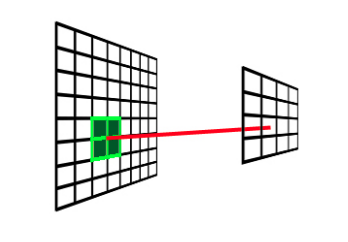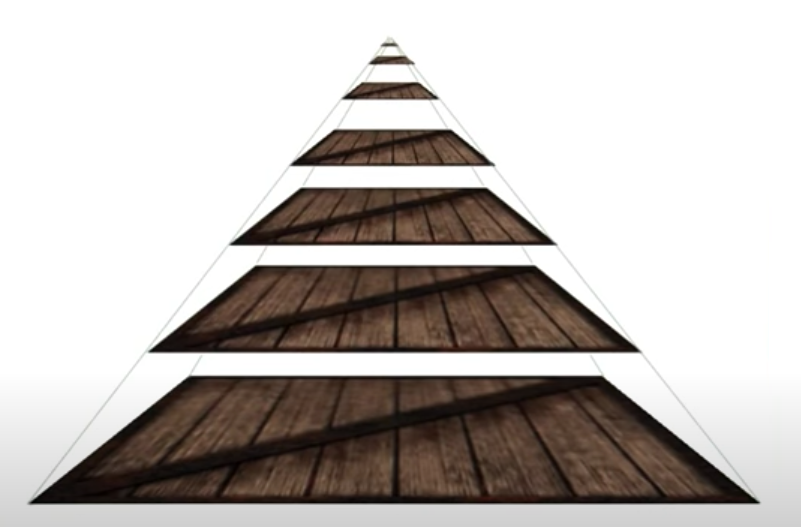• 当一个像素覆盖了 1 个纹素的时候，采样时应该访问第 0 层 mipmap
• 当一个像素覆盖了 2 个纹素的时候，采样时应该访问第 1 层 mipmap
• 当一个像素覆盖了 4 个纹素的时候，采样时应该访问第 2 层 mipmap
• 当一个像素覆盖了 128 个纹素的时候，采样时应该访问第 7 层 mipmap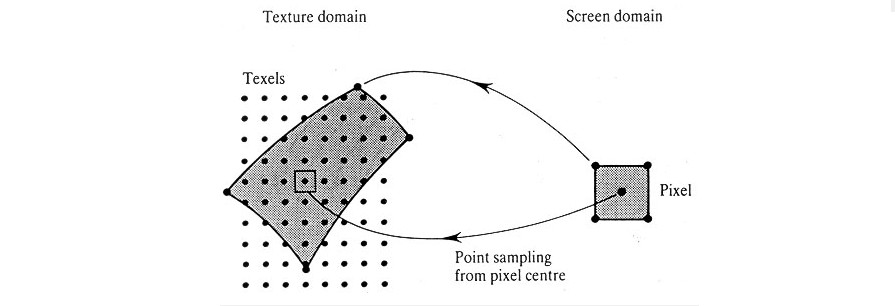$$L = Log_2 N$$

$$S_n = a_1 \frac {1 - q^n} {1 - q}$$

$$S_n = 1 \cdot \frac {1 - {\frac 1 4^n}} {\frac 3 4} = \frac 4 3 \cdot (1 - \frac 1 4^n)$$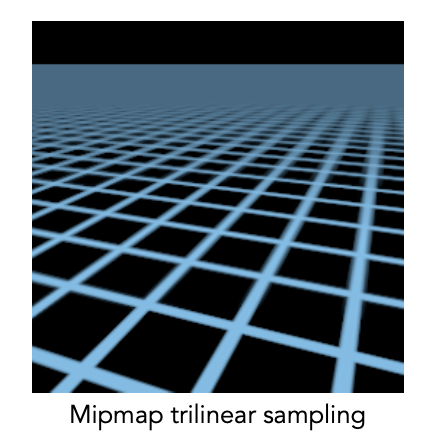## 各向异性过滤（Anisotropic Filtering）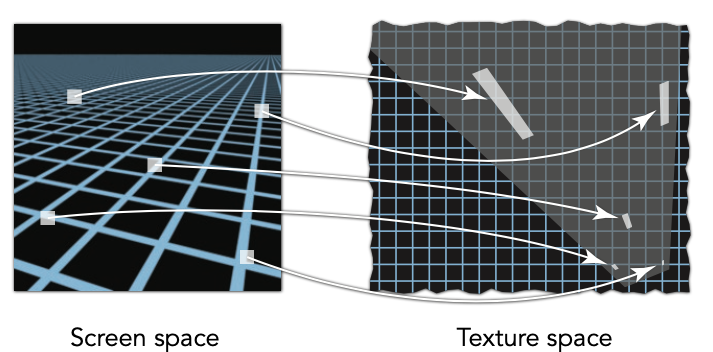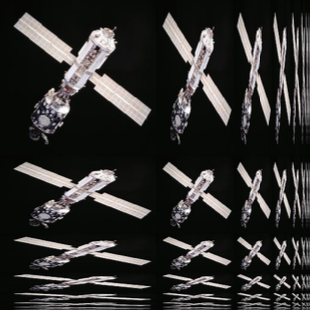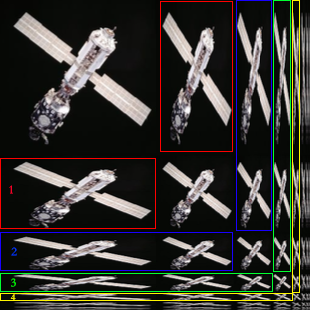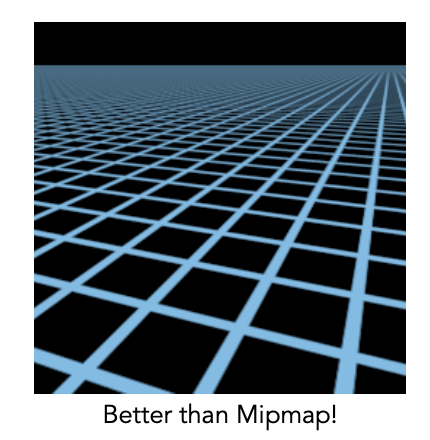# 各式各样的纹理

## 球面贴图（Spherical Map）## 立方体贴图（Cube Map）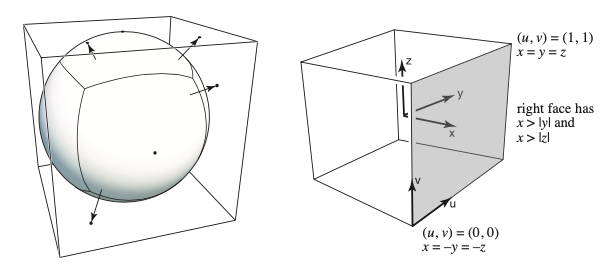## 凹凸贴图（Bump Map）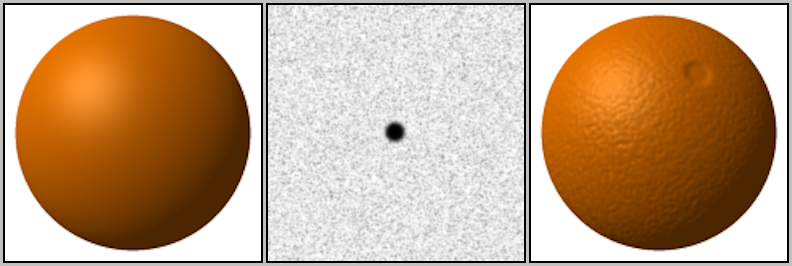## 法线贴图（Normal Map）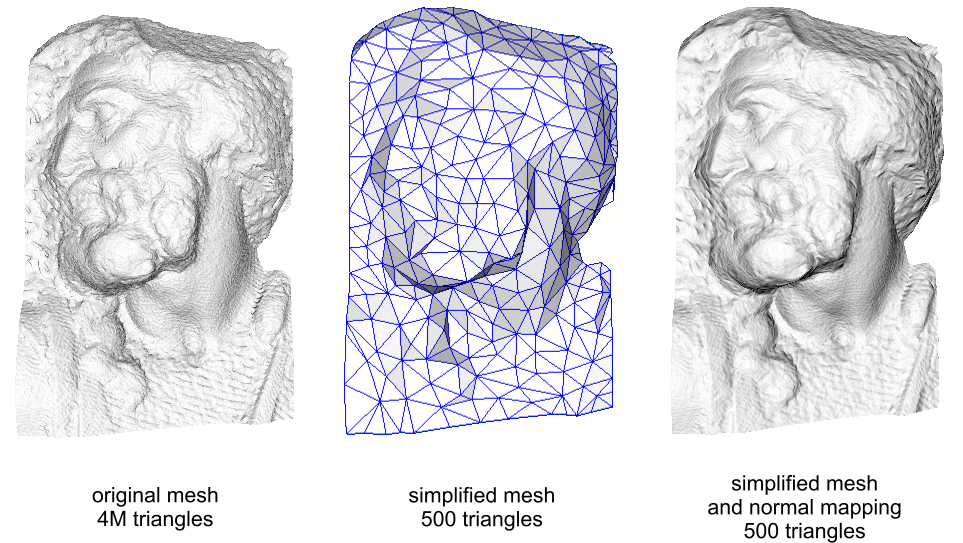# 作业

## 参数插值

  1 2 3 4 5 6 7 8 9 10 11 12 13 14 15 16 17 18 19 20 21 22 23 24 25 26 27 28 29 30 31 32 33 34 35 36 37 38 39 40 41 42 43 44 45 46 47 48 49 50 51  // rasterizer.cpp void rst::rasterizer::rasterize_triangle(const Triangle& t, const std::array& view_pos) { auto v = t.toVector4(); int top = ceil(std::max(v.y(), std::max(v.y(), v.y()))); int bottom = floor(std::min(v.y(), std::min(v.y(), v.y()))); int left = floor(std::min(v.x(), std::min(v.x(), v.x()))); int right = ceil(std::max(v.x(), std::max(v.x(), v.x()))); for (int i = 0; i < 3; i++) { Eigen::Vector4f vertex = v[i]; float x = vertex.x(); float y = vertex.y(); if (y > top) top = ceil(y); if (y < bottom) bottom = floor(y); if (x > right) right = ceil(x); if (x < left) left = floor(x); } for (int x = left; x < right; x++) { for (int y = bottom; y < top; y++) { if (insideTriangle(x, y, t.v)) { auto[alpha, beta, gamma] = computeBarycentric2D(x, y, t.v); float Z = 1.0 / (alpha / v.w() + beta / v.w() + gamma / v.w()); float zp = alpha * v.z() / v.w() + beta * v.z() / v.w() + gamma * v.z() / v.w(); zp *= Z; int index = get_index(x, y); if (depth_buf[index] > zp) { depth_buf[index] = zp; auto interpolated_color = interpolate(alpha, beta, gamma, t.color, t.color, t.color, 1); auto interpolated_normal = interpolate(alpha, beta, gamma, t.normal, t.normal, t.normal, 1); auto interpolated_texcoords = interpolate(alpha, beta, gamma, t.tex_coords, t.tex_coords, t.tex_coords, 1); auto interpolated_shadingcoords = interpolate(alpha, beta, gamma, view_pos, view_pos, view_pos, 1); // fragment_shader_payload payload(interpolated_color, interpolated_normal.normalized(), interpolated_texcoords, texture ? &*texture : nullptr); payload.view_pos = interpolated_shadingcoords; auto pixel_color = fragment_shader(payload); set_pixel(Eigen::Vector2i(x, y), pixel_color); } } } } } 

## Blinn-Phong 反射模型

  1 2 3 4 5 6 7 8 9 10 11 12 13 14 15 16 17 18 19 20 21 22 23 24 25 26 27 28 29 30 31 32 33 34 35 36 37 38 39 40 41 42  // main.cpp Eigen::Vector3f phong_fragment_shader(const fragment_shader_payload& payload) { Eigen::Vector3f ka = Eigen::Vector3f(0.005, 0.005, 0.005); Eigen::Vector3f kd = payload.color; Eigen::Vector3f ks = Eigen::Vector3f(0.7937, 0.7937, 0.7937); auto l1 = light{{20, 20, 20}, {500, 500, 500}}; auto l2 = light{{-20, 20, 0}, {500, 500, 500}}; std::vector lights = {l1, l2}; Eigen::Vector3f amb_light_intensity{10, 10, 10}; Eigen::Vector3f eye_pos{0, 0, 10}; float p = 150; Eigen::Vector3f color = payload.color; Eigen::Vector3f point = payload.view_pos; Eigen::Vector3f normal = payload.normal; Eigen::Vector3f result_color = {0, 0, 0}; Eigen::Vector3f La = ka.cwiseProduct(amb_light_intensity); result_color += La; for (auto& light : lights) { Eigen::Vector3f view_dir = (eye_pos - point).normalized(); Eigen::Vector3f light_dir = (light.position - point).normalized(); Eigen::Vector3f half_dir = (view_dir + light_dir).normalized(); Eigen::Vector3f point_to_light = light.position - point; double r = point_to_light.dot(point_to_light); double NoL = std::max(0.0f, normal.normalized().dot(light_dir)); double VoH = std::max(0.0f, normal.normalized().dot(half_dir)); Eigen::Vector3f Ld = kd.cwiseProduct(light.intensity / r) * NoL; Eigen::Vector3f Ls = ks.cwiseProduct(light.intensity / r) * std::pow(VoH, p); result_color += (Ld + Ls); } return result_color * 255.f; } 

## Texture mapping

  1 2 3 4 5 6 7 8 9 10 11 12 13 14 15 16 17 18 19 20 21 22 23 24 25 26 27 28 29 30 31 32 33 34 35 36 37 38 39 40 41 42 43 44 45 46 47 48 49  // main.cpp Eigen::Vector3f texture_fragment_shader(const fragment_shader_payload& payload) { Eigen::Vector3f return_color = {0, 0, 0}; if (payload.texture) { return_color = payload.texture->getColor(payload.tex_coords.x(), payload.tex_coords.y()); } Eigen::Vector3f texture_color; texture_color << return_color.x(), return_color.y(), return_color.z(); Eigen::Vector3f ka = Eigen::Vector3f(0.005, 0.005, 0.005); Eigen::Vector3f kd = texture_color / 255.f; Eigen::Vector3f ks = Eigen::Vector3f(0.7937, 0.7937, 0.7937); auto l1 = light{{20, 20, 20}, {500, 500, 500}}; auto l2 = light{{-20, 20, 0}, {500, 500, 500}}; std::vector lights = {l1, l2}; Eigen::Vector3f amb_light_intensity{10, 10, 10}; Eigen::Vector3f eye_pos{0, 0, 10}; float p = 150; Eigen::Vector3f color = texture_color; Eigen::Vector3f point = payload.view_pos; Eigen::Vector3f normal = payload.normal; Eigen::Vector3f result_color = {0, 0, 0}; Eigen::Vector3f La = ka.cwiseProduct(amb_light_intensity); result_color += La; for (auto& light : lights) { Eigen::Vector3f view_dir = (eye_pos - point).normalized(); Eigen::Vector3f light_dir = (light.position - point).normalized(); Eigen::Vector3f half_dir = (view_dir + light_dir).normalized(); Eigen::Vector3f point_to_light = light.position - point; double r = point_to_light.dot(point_to_light); double NoL = std::max(0.0f, normal.normalized().dot(light_dir)); double VoH = std::max(0.0f, normal.normalized().dot(half_dir)); Eigen::Vector3f Ld = kd.cwiseProduct(light.intensity / r) * NoL; Eigen::Vector3f Ls = ks.cwiseProduct(light.intensity / r) * std::pow(VoH, p); result_color += (Ld + Ls); } return result_color * 255.f; } 

## Bump mapping

  1 2 3 4 5 6 7 8 9 10 11 12 13 14 15 16 17 18 19 20 21 22 23 24 25 26 27 28 29 30 31 32 33 34 35 36 37 38 39 40 41 42 43 44 45 46 47 48 49  // main.cpp Eigen::Vector3f bump_fragment_shader(const fragment_shader_payload& payload) { Eigen::Vector3f ka = Eigen::Vector3f(0.005, 0.005, 0.005); Eigen::Vector3f kd = payload.color; Eigen::Vector3f ks = Eigen::Vector3f(0.7937, 0.7937, 0.7937); auto l1 = light{{20, 20, 20}, {500, 500, 500}}; auto l2 = light{{-20, 20, 0}, {500, 500, 500}}; std::vector lights = {l1, l2}; Eigen::Vector3f amb_light_intensity{10, 10, 10}; Eigen::Vector3f eye_pos{0, 0, 10}; float p = 150; Eigen::Vector3f color = payload.color; Eigen::Vector3f point = payload.view_pos; Eigen::Vector3f normal = payload.normal; float kh = 0.2, kn = 0.1; float x = normal.x(); float y = normal.y(); float z = normal.z(); float u = payload.tex_coords.x(); float v = payload.tex_coords.y(); float w = payload.texture->width; float h = payload.texture->height; Eigen::Vector3f t(x * y / std::sqrt(x * x + z * z), std::sqrt(x * x + z * z), z * y / std::sqrt(x * x + z * z)); Eigen::Vector3f b = normal.cross(t); Eigen::Matrix3f TBN; TBN << t, b, normal; float dU = kh * kn * (payload.texture->getColor(u + 1.0f / w, v).norm() - payload.texture->getColor(u, v).norm()); float dV = kh * kn * (payload.texture->getColor(u, v + 1.0f / h).norm() - payload.texture->getColor(u, v).norm()); Eigen::Vector3f ln(-dU, -dV, 1.0); normal = TBN * ln; normal.normalized(); Eigen::Vector3f result_color = normal; return result_color * 255.f; } 

## Displacement mapping

  1 2 3 4 5 6 7 8 9 10 11 12 13 14 15 16 17 18 19 20 21 22 23 24 25 26 27 28 29 30 31 32 33 34 35 36 37 38 39 40 41 42 43 44 45 46 47 48 49 50 51 52 53 54 55 56 57 58 59 60 61 62 63 64 65 66  // main.cpp Eigen::Vector3f displacement_fragment_shader(const fragment_shader_payload& payload) { Eigen::Vector3f ka = Eigen::Vector3f(0.005, 0.005, 0.005); Eigen::Vector3f kd = payload.color; Eigen::Vector3f ks = Eigen::Vector3f(0.7937, 0.7937, 0.7937); auto l1 = light{{20, 20, 20}, {500, 500, 500}}; auto l2 = light{{-20, 20, 0}, {500, 500, 500}}; std::vector lights = {l1, l2}; Eigen::Vector3f amb_light_intensity{10, 10, 10}; Eigen::Vector3f eye_pos{0, 0, 10}; float p = 150; Eigen::Vector3f color = payload.color; Eigen::Vector3f point = payload.view_pos; Eigen::Vector3f normal = payload.normal; float kh = 0.2, kn = 0.1; float x = normal.x(); float y = normal.y(); float z = normal.z(); float u = payload.tex_coords.x(); float v = payload.tex_coords.y(); float w = payload.texture->width; float h = payload.texture->height; Eigen::Vector3f t(x * y / std::sqrt(x * x + z * z), std::sqrt(x * x + z * z), z * y / std::sqrt(x * x + z * z)); Eigen::Vector3f b = normal.cross(t); Eigen::Matrix3f TBN; TBN << t, b, normal; float dU = kh * kn * (payload.texture->getColor(u + 1.0f / w, v).norm() - payload.texture->getColor(u, v).norm()); float dV = kh * kn * (payload.texture->getColor(u, v + 1.0f / h).norm() - payload.texture->getColor(u, v).norm()); Eigen::Vector3f ln(-dU, -dV, 1.0); point = point + kn * normal * payload.texture->getColor(u, v).norm(); normal = TBN * ln; normal = normal.normalized(); Eigen::Vector3f result_color = {0, 0, 0}; Eigen::Vector3f La = ka.cwiseProduct(amb_light_intensity); result_color += La; for (auto& light : lights) { Eigen::Vector3f view_dir = (eye_pos - point).normalized(); Eigen::Vector3f light_dir = (light.position - point).normalized(); Eigen::Vector3f half_dir = (view_dir + light_dir).normalized(); Eigen::Vector3f point_to_light = light.position - point; double r = point_to_light.dot(point_to_light); double NoL = std::max(0.0f, normal.normalized().dot(light_dir)); double VoH = std::max(0.0f, normal.normalized().dot(half_dir)); Eigen::Vector3f Ld = kd.cwiseProduct(light.intensity / r) * NoL; Eigen::Vector3f Ls = ks.cwiseProduct(light.intensity / r) * std::pow(VoH, p); result_color += (Ld + Ls); } return result_color * 255.f; } 

# 参考资料

Lecture 09 Shading 3 (Texture Mapping Cont.)

Lecture 10 Geometry 1 (Introduction)

Pixel vs. Texel

Textures - Magnification and Minification

MipMap Texturing

Mipmapping

Linear Filtering

Needs More Pictures